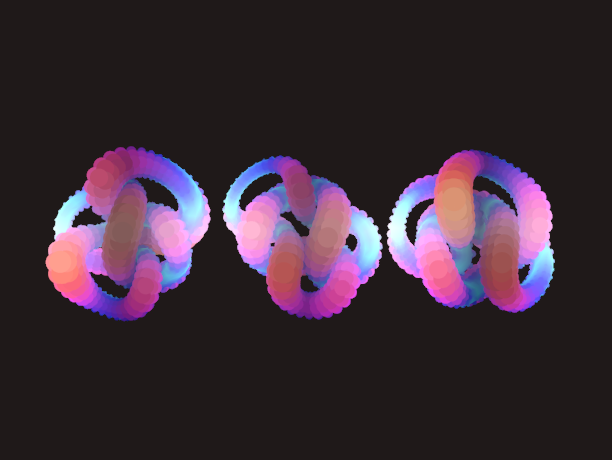knots

➰ A program for manipulating and playing with knot diagrams.Description

Grid Diagrams

One interesting way of representing a knot is via a so-called nxn grid diagram. Each cell of the grid is either an x, an o, or "blank." Each row and column is restricted to have exactly one x and one o. We can construct the knot corresponding to a particular grid diagram using the following procedure:

1. In each column, connect each x to the corresponding o
2. In each row, connect each o to the corresponding x
3. Whenever a horizontal segment intersects a vertical segment, assume that the vertical segment passes over the horizontal segment (i.e. a grid diagram only consists of over-crossings)

Refinement

Following the procedure outlined above results in a piecewise linear link (polyline). To obtain a "smoother" projection of the knot (one without sharp corners), we need to perform some form of topological refinement, taking care to not change the underlying structure of the knot. Following Dr. Scharein's thesis (link below), each vertex of the generated polyline is treated as a particle in a physics simulation. Adjacent vertices are attracted to one another via a mechanical spring force. Non-adjacent particles are repelled from one another via an electrostatic force, which (in practice) prevents segments from crossing over or under one another. A more robust method would perform intersection tests between all pairs of non-neighboring line segments to prevent any "illegal" crossings. This is currently a TODO item.

In order for this to work, the polyline must start in a "valid" state, where no two line segments intersect. To accomodate this, crossings are calculated based on criteria (3) above. A new vertex is added at each point of crossing and "lifted" along the z-axis by some small amount. The entire polyline is then resampled, to increase vertex density.

Before the knot is rendered, a path-guided extrusion is performed to "thicken" the knot. At each vertex along the polyline, a coordinate frame is established by calculating the tangent vector and a vector orthogonal to the tangent. Then, a circular cross-section is added at the origin of this new, local coordinate system. Adjacent cross-sections are connected with triangles to form a continuous, closed "tube." To avoid jarring rotations, parallel transport is employed. Essentially, each successive coordinate frame is calculated with respect to the previous frame. This ensures that the circular cross-sections smoothly rotate around the polyline during traversal.

Cromwell Moves

The Cromwell Moves are similar to the Reidemeister Moves, specifically applied to grid diagrams. They all us to obtain isotopic knots, i.e. knots that have the same underlying topology but "look" different. This gives us a way to systematically explore a given knot invariant.

In knots, the Cromwell Moves are represented as an enum:

enum CromwellMove {
// A move that cyclically translates a row or column in one of four directions: up, down, left, or right
Translation(Direction),

// A move that exchanges to adjacent, non-interleaved rows or columns
Commutation { axis: Axis, start_index: usize, },

// A move that replaces an `x` with a 2x2 sub-grid
Stabilization { cardinality: Cardinality, i: usize, j: usize, },

// A move that replaces a 2x2 sub-grid with an `x` (the opposite of an x-stabilization): currently not supported
Destabilization { cardinality: Cardinality, i: usize, j: usize, },
}

Tested On

• Windows 8.1
• NVIDIA GeForce GT 750M
• Rust compiler version 1.38.0-nightly (nightly may not be required)

NOTE: this project will only run on graphics cards that support OpenGL Direct State Access (DSA).

To Build

1. Clone this repo.
2. Make sure 🦀 Rust installed and cargo is in your PATH.
3. Inside the repo, run: cargo build --release.

To Use

All grid diagrams must be "square" .csv files (the same number of rows as columns). Each row and column must have exactly one x and one o: all other entries should be spaces ("blank"). The grid diagram will be validated upon construction, but the program will panic! if one of the conditions above is not met. An example grid diagram for the trefoil knot is shown below:

"x"," ","o"," "," "
" ","x"," ","o"," "
" "," ","x"," ","o"
"o"," "," ","x"," "
" ","o"," "," ","x"

To rotate the camera around the object in 3-dimensions, press + drag the left mouse button. Press h to "home" (i.e. reset) the camera.

You can change between wireframe and filled modes by pressing w and f. You can save out a screenshot by pressing s. Finally, you can reset the physics simulation by pressing r.

To Do

• [ ] Implement a knot "drawing" tool
• [ ] Add segment-segment intersection test for more robust topological refinement
• [ ] Add a GUI for viewing the current grid diagram
• [ ] Generate the Dowker notation for a given knot diagram
• [ ] Explore planar graphs and their relationship to knot diagrams
• [ ] Implement a tangle calculator for generating knots (Conway notation)

Credits

This project was largely inspired by and based on previous work done by Dr. Robert Scharein, whose PhD thesis and software were vital towards my understanding of the relaxation / meshing procedures.